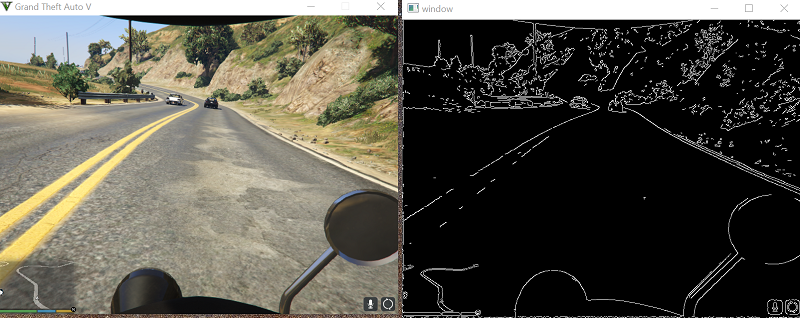OpenCV basics - Python Plays GTA V

pygta5-2-OpenCV-basics

Now that we're confident that we can at least get the screen data, the next thing we want to do is run some OpenCV operations on the data that are typical for vision tasks.

For example, we'll want to conver the image to grayscale to simplify things (one value vs RGB's 3 values per pixels) and edge detection to eventually be used for finding the lines that will be our lanes.

The code up to this point:

import numpy as np
from PIL import ImageGrab
import cv2
import time

# just so this doesn't go on forever:

def screen_record():
last_time = time.time()
while(True):
# 800x600 windowed mode
printscreen =  np.array(ImageGrab.grab(bbox=(0,40,800,640)))
print('loop took {} seconds'.format(time.time()-last_time))
last_time = time.time()
cv2.imshow('window',cv2.cvtColor(printscreen, cv2.COLOR_BGR2RGB))
if cv2.waitKey(25) & 0xFF == ord('q'):
cv2.destroyAllWindows()
break

#screen_record()

Now let's add some grayscale and edges:

import numpy as np
from PIL import ImageGrab
import cv2
import time

def process_img(image):
original_image = image
# convert to gray
processed_img = cv2.cvtColor(image, cv2.COLOR_BGR2GRAY)
# edge detection
processed_img =  cv2.Canny(processed_img, threshold1 = 200, threshold2=300)
return processed_img

def main():
last_time = time.time()
while True:
screen =  np.array(ImageGrab.grab(bbox=(0,40,800,640)))
#print('Frame took {} seconds'.format(time.time()-last_time))
last_time = time.time()
new_screen = process_img(screen)
cv2.imshow('window', new_screen)
#cv2.imshow('window',cv2.cvtColor(screen, cv2.COLOR_BGR2RGB))
if cv2.waitKey(25) & 0xFF == ord('q'):
cv2.destroyAllWindows()
break
#main()
from IPython.display import Image
Image(filename='edge-detection.png')Looking great!

In my excitement of getting the screen recording working, I haven't yet tested PyAutoGUI, so we can do that next.

The next tutorial:• Reading game frames in Python with OpenCV - Python Plays GTA V

• OpenCV basics - Python Plays GTA V
• Direct Input to Game - Python Plays GTA V

• Region of Interest for finding lanes - Python Plays GTA V

• Hough Lines - Python Plays GTA V

• Finding Lanes for our self driving car - Python Plays GTA V

• Self Driving Car - Python Plays GTA V

• Next steps for Deep Learning self driving car - Python Plays GTA V

• Training data for self driving car neural network- Python Plays GTA V

• Balancing neural network training data- Python Plays GTA V

• Training Self-Driving Car neural network- Python Plays GTA V

• Testing self-driving car neural network- Python Plays GTA V

• A more interesting self-driving AI - Python Plays GTA V

• Object detection with Tensorflow - Self Driving Cars in GTA

• Determining other vehicle distances and collision warning - Self Driving Cars in GTA

• Getting the Agent a Vehicle- Python Plays GTA V

• Acquiring a Vehicle for the Agent - Python Plays GTA V